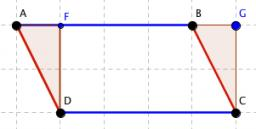# Parallelogram 6049

Calculate the area of the parallelogram if a = 57cm, the diagonal u = 66cm, and the angle against the diagonal is beta β = 57° 43'

S =  3640.2865 cm2

### Step-by-step explanation:Did you find an error or inaccuracy? Feel free to write us. Thank you!

Tips for related online calculators
Need help calculating sum, simplifying, or multiplying fractions? Try our fraction calculator.